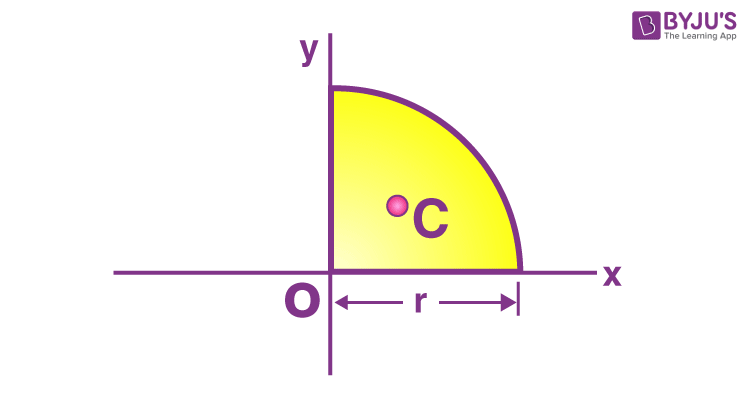Checkout JEE MAINS 2022 Question Paper Analysis : Checkout JEE MAINS 2022 Question Paper Analysis :

# Moment Of Inertia Of A Quarter Circle

Moment of inertia of a quarter circle is usually found or calculated using the given formula;

 I = π R4 / 16

In this lesson, we will learn how to derive the formula as well as its application in problems.

## Moment Of Inertia Of Quarter Circle Derivation

When we are deriving the moment of inertia expression for a quarter circle, we can partly use the same derivation that is followed for determining the moment of inertia of a circle. The concept is more or less the same.1. We will first have a look at a full circle formula. It is given as;

I = πr4 / 4

If we want to derive the equation for a quarter circle then we basically have to divide the results obtained for a full circle by two and get the result for a quarter circle. Notably, in a full circle, the moment of inertia relative to the x-axis is the same as the y-axis.

With that concept we get;

Ix = Iy = ¼ πr4

Jo = Ix + Iy = ¼ πr4 + ¼ πr4 = ½ πr4

We will need to determine the area of a circle as well. When we are solving this expression we usually replace M with Area, A.

Jo = ½ (πr2) R2

Now if take a quarter circle, the moment of inertia relative to the x-axis and y-axis will be one quarter the moment inertia of a full circle. However, the part of the circle rotating about an axis will be symmetric and the values will be equal for both the y and x-axis. With that, we will solve the equation below.

Ix = Iy = 1/16 πr4

= 1/16 (πr2) R2

= 1 /16 (A) R2

= ¼ (¼ Ao) R2

The next step involves finding the moment of inertia of a quarter circle. For this, we will simply add the values of both x and y-axis.

M.O.I relative to the origin, Jo = Ix + Iy

= 1 / 16 (A)R2 + 1 / 16 (A)R2

= ⅛ (A)R2

= ⅛ (πr2)R2

= ⅛ πr4

## Parallel Axis Theorem